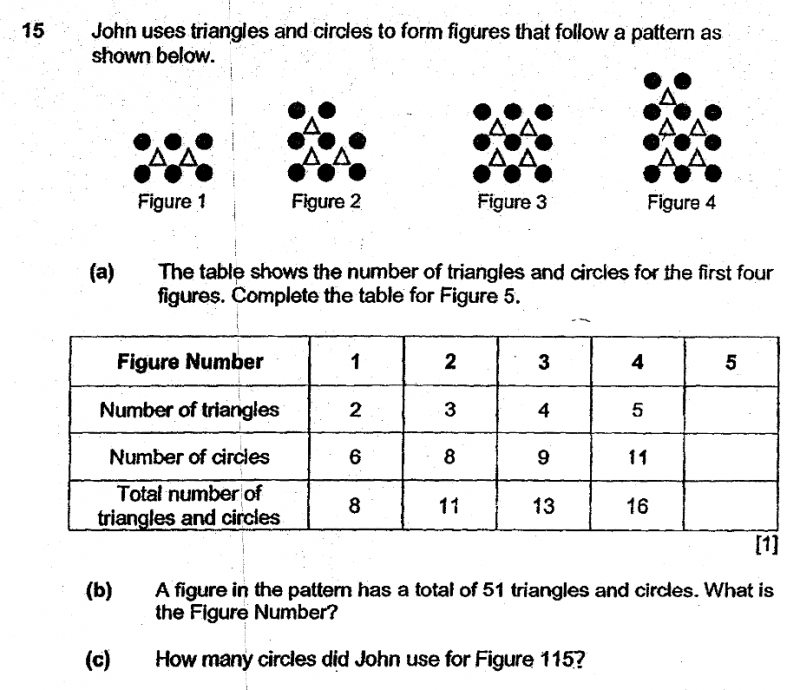# QuestionHi KP’s,

Thanks & Regards,

DNLNMUM

a)

 Figure Number 1 2 3 4 5 No. of triangles 2 3 4 5 6 No. of circles 6 8 9 11 12 Total no. of shapes 8 11 13 16 18

b) Looking at the last row for the total number of shapes, we can see that the pattern is +3, +2, +3, +2… if we group a +3 and a +2 together, we get a +5.

The figure we want to get to is 51 and we already have 8 as a start so we need to find out how many groups of +5 is needed to get there.

First, we take 51 – 8 = 43
43 ÷ 5 = 8 r 3

This means we need 8 groups of +5 or (+2 & +3) and an additional +3 to get to 51.

8 groups of +5 is basically 8 x 2 = 16 patterns
We then add on the first pattern and the last pattern (remainder of +3).

Therefore, the 18th pattern will have 51 shapes.

c) To find the no. of circles, we can take the following steps:

1. Add 2 to the quotient of (Figure Number ÷ 2), ignoring any remainder (this is to find the number of rows of circles)
2. Multiply the sum by 3 (3 circles per row)
3. If the Figure Number is an even number, subtract 1 (as there will be a missing circle at the top right hand corner)

115 ÷ 2 = 57 r 1
57 + 2 = 59
59 x 3 = 177 circles